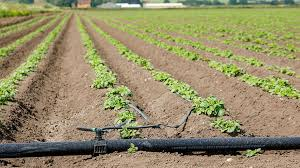## How to Calculate and Solve for Application Efficiency for Trickle Irrigation | Irrigation Water RequirementThe image above represents application efficiency for trickle irrigation.

To compute for application efficiency for trickle irrigation, two essential parameters are needed and these parameters are Emission Uniformity (Euand Application Efficiency for Sprinkler (Ea).

The formula for calculating application efficiency for trickle irrigation:

Eta = Eu x Ea x 100

Where:

Eta = Application Efficiency for Trickle Irrigation
Eu = Emission Uniformity
Ea = Application Efficiency for Sprinkler

Let’s solve an example;
Find the application efficiency for trickle irrigation when the emission uniformity is 32 and the applicatio efficiency for sprinkler is 4.

This implies that;

Eu = Emission Uniformity = 32
Ea = Application Efficiency for Sprinkler = 4

Eta = Eu x Ea x 100
Eta = (32) x (4) x 100
Eta = 12800

Therefore, the application efficiency for trickle irrigation is 12800%.

Calculating for the Emission Uniformity when the Application Efficiency for Trickle Irrigation and the Application Efficiency for Sprinkler is Given.

Eu = Eta / Ea x 100

Where;

Eu = Emission Uniformity
Eta = Application Efficiency for Trickle Irrigation
Ea = Application Efficiency for Sprinkler

Let’s solve an example;
Find the emission uniformity when the application efficiency for trickle irrigation is 60 and the application efficiency for sprinkler is 2.

This implies that;

Eta = Application Efficiency for Trickle Irrigation = 60
Ea = Application Efficiency for Sprinkler = 2

Eu = Eta / Ea x 100
Eu = 60 / 2 x 100
Eu = 60 / 200
Eu = 0.3

Therefore, the emission uniformity is 0.3%.